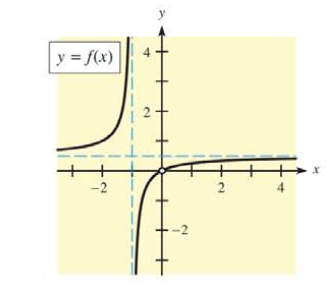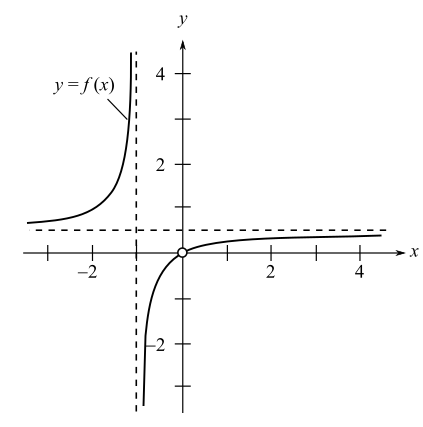Chapter 9, Problem 36RE### Mathematical Applications for the ...

11th Edition
Ronald J. Harshbarger + 1 other
ISBN: 9781305108042

#### Solutions

Chapter
Section### Mathematical Applications for the ...

11th Edition
Ronald J. Harshbarger + 1 other
ISBN: 9781305108042
Textbook Problem

# In Problems 35 and 36, use the graphs to find (a) the points of discontinuity, (b) lim x → ∞ f ( x ) , and (c) lim x → − ∞ f ( x ) .(a)

To determine

The points of continuity for the function with the graph,Explanation

Given Information:

The provided graph of the function is,

Explanation:

Consider the provided graph of the function,

For a function to be continuous at x=a the left hand limit must be equal to the right hand limit and the value of the function at x=a must be equal to the limits.

From the graph, the value of the function does not exist at x=0, as the point is indicated with a hollow circle. Also, the value of the function approaching from the left is 0 and the right is approaching 0 that are equal, but the value of the function does not exist at x=0

(b)

To determine

The value of limxf(x) from the graph of the function,(c)

To determine

The value of limxf(x) from the graph of the function,### Still sussing out bartleby?

Check out a sample textbook solution.

See a sample solution

#### The Solution to Your Study Problems

Bartleby provides explanations to thousands of textbook problems written by our experts, many with advanced degrees!

Get Started

#### Evaluate the expression sin Exercises 116. 2322

Finite Mathematics and Applied Calculus (MindTap Course List)

#### Divide: (72x3yz4)(162xy2)

Elementary Technical Mathematics

#### An estimate of 13x4 using Simpsons Rule with n = 4 gives: a) 2425 b) 1483 c) 1523 d) 2445

Study Guide for Stewart's Single Variable Calculus: Early Transcendentals, 8th